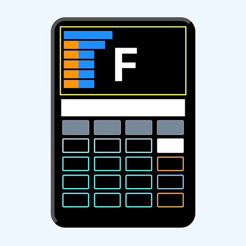## Screenshots

••••••••••## Description

UtilMatF is a financial calculator that, due to its power of calculations and graphical possibilities, presents itself as a great tool for working, studying and teaching financial mathematics.

The ability to show how financial calculations are achieved through tables in most cases (Dsp), as well as converting them into graphics and being able to expand them even on a special screen, in order to visualize the effects of a given action makes it in an ideal financial tool.

Financial functions include:

Simple interest
Compound interest
- Savings
- Installment savings
- Loans
Amortization of loans
Investment evaluation
Bond Quote Calculations
Depreciation
Cost, sales price and profit margin calculations
Conversion of interest
- Conversion of percentage interest rate (APR) to effective interest rate (EFF)
- Conversion of effective interest rate (EFF) to percentage interest rate (APR)
Calculations with dates

Three examples are included in the "particularities" area. One of compound interest calculations and two of bond calculations.

Most financial operations include a development table so that the calculation can be viewed and analyzed (Dsp).

Financial variable memories can be switched between operations to facilitate analysis. Also, these data can be visualized or rescued for use (MEM) in mathematical calculations in case an additional special calculation is required.

Graphics (G) (Gra):

The conversion of the calculations made into graphs and that the options presented can highlight or make the effects of the variables independent, make it present as a great financial tool.

In mathematical operations, other than financial, the following are included:

Nth exponent
Square root or cubic root
Power of e, power of ten
Neperian and decimal logarithm
Inverse and factorial function
Simple rule of three - Direct
Simple Rule of Three - Inverse
Interpolation

The visualization of the operations is done online allowing to review the data entry.

It is feasible to rescue the last operation (as I am satisfied) in order to review the operation.

A Particularities area of ​​this financial tool is included, which together with its message and alert system make this tool very friendly.

## Information

Seller
Victor Hidalgo
Size
10.1 MB
Category
Education
Compatibility

Requires iOS 10.0 or later. Compatible with iPhone, iPad, and iPod touch.

Languages

English

Age Rating
4+
•